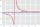# Inequation

Solve the inequation:

5k - (7k - 1)≤ 2/5 . (5-k)-2

Result

k≥:  0.625

#### Solution:Leave us a comment of example and its solution (i.e. if it is still somewhat unclear...):Be the first to comment!## Next similar examples:

1. InqualitySolve inequality: 3x + 6 > 14
2. EquationSolve the equation: 1/2-2/8 = 1/10; Write the result as a decimal number.
3. ReciprocalIt is true (prove it) that if a> b> 0: ?
4. Simplify 2Simplify expression: 5ab-7+3ba-9
5. Unknown numberIdentify unknown number which 1/5 is 40 greater than one tenth of that number.
6. Percents - easyHow many percent is 432 out of 434?
7. GlovesI have a box with two hundred pieces of gloves in total, split into ten parcels of twenty pieces, and I sell three parcels. What percent of the total amount I sold?
8. MO 2016 Numerical axisCat's school use a special numerical axis. The distance between the numbers 1 and 2 is 1 cm, the distance between the numbers 2 and 3 is 3 cm, between the numbers 3 and 4 is 5 cm and so on, the distance between the next pair of natural numbers is always in
9. Sales offGoods is worth € 70 and the price of goods fell two weeks in a row by 10%. How many % decreased overall?
10. The percentages in practiceIf every tenth apple on the tree is rotten it can be expressed by percentages: 10% of the apples on the tree is rotten. Tell percent using the following information: a. in June rained 6 days b, increase worker pay 500 euros to 50 euros c, grabbed 21 fro
11. Down syndromeDown syndrome is one of the serious diseases caused by a gene mutation. Down syndrome occurs in approximately every 550-born child. Express the incidence of Down's syndrome in newborns at per mille.
12. NumberWhat number is 20 % smaller than the number 198?
13. TVsProduction of television sets increased from 3,500 units to 4,200 units. Calculate the percentage of production increase.
14. Base, percents, valueBase is 344084 which is 100 %. How many percent is 384177?
15. Conference148 is the total number of employees. The conference was attended by 22 employees. How much is it in percent?
16. ClassIn a class are 32 pupils. Of these are 8 boys. What percentage of girls are in the class?
17. Apples 2James has 13 apples. He has 30 percent more apples than Sam. How many apples has Sam?